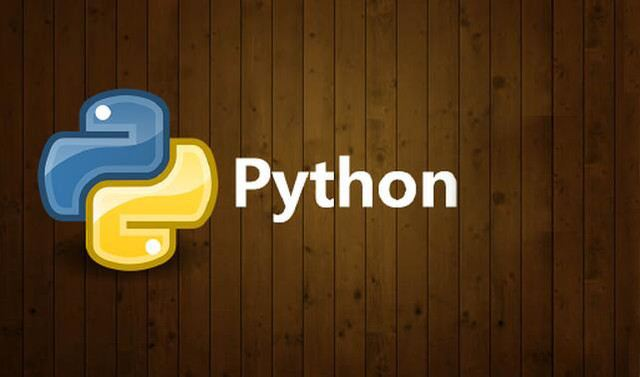#Python为什么流行？Python代码能实现哪些有趣的功能

0
0
01. 云栖社区>
2. 博客>
3. 正文

## Python为什么流行？Python代码能实现哪些有趣的功能

python究竟有多强大？Python代码能实现哪些功能？众所周知，Python入门简单、功能强大，是人工智能时代最佳的编程语言。但很多人好奇Python究竟有多强大，为什么那么受欢迎?下面就来给大家揭晓答案，看一下一行Python代码究竟能实现哪些功能!Python为什么流行？Python代码能实现哪些有趣的功能
1、九九乘法表

print('

'.join([' '.join(['%s%s=%-2s' % (y, x, xy) for y in range(1, x+1)]) for x in range(1, 10)]))

2、打印心形图案

print('

'.join([''.join([('AndyLove'[(x-y)%8]if((x0.05)2+(y0.1)2-1)3-(x0.05)2(y0.1)*3<=0 else' ')for x in range(-30,30)])for y in range(15,-15,-1)]))

3、动态螺旋桨

exec("""

from turtle import *

for i in range(500):

forward(i)

left(91)

""")

4、计算1-100以内的素数

print(' '.join([str(item) for item in filter(lambda x: not [x % i for i in range(2, x) if x % i == 0], range(2, 101))]))

5、输出斐波那契数列

print([x for x in (a[i, a.append(a[i, ai+ai])) for a in ([[1, 1]] ) for i in range(30)]])

6、实现快排算法

quickSort = lambda array: array if len(array) <= 1 else quickSort([item for item in array[1:] if item <= array]) + [array] + quickSort([item for item in array[1:] if item > array])

array = [9, 11, 88, 32, 8]

print(quickSort(array))

7、单线迷宫

cmd 命令下输入下列代码实现单线迷宫。

python -c "while 1:import random;print(random.choice('|| __'), end='')"

8、求解2的1000次方的各位数之和

2的1000次方的值很大，把结果每位数都加起来等于多少呢?

print(sum(map(int, str(2**1000))))# 结果 13661)Scrapy。Scrapy是一个为了爬取网站数据、提取结构性数据而编写的应用框架，可以应用在数据挖掘，信息处理或存储历史数据等一系列的程序中。Scrapy用途广泛，可以用于数据挖掘、监测和自动化测试。

2)Numpy。Numpy被认为是Python中最流行的机器学习库之一，具有很强的交互性和易用性，能够使复杂的数学实现非常简单。Numpy接口可用于将图像、声波和其他二进制原始流表示为N维中的实数数组。

3)Keras。Keras被认为是Python中最酷的机器学习库之一。它提供了一种更容易表达神经网络的机制。Keras还为编译模型、处理数据集、图形可视化等提供了一些最好的实用工具。

4)Pandas。Pandas是Python中的机器学习库，它提供高层次的数据结构和各种各样的分析工具，这个库最大的特点之一是能够使用一两条命令来转换带有数据的复杂操作。Pandas有许多内置的方法来分组、合并数据、过滤，以及时间序列功能。

+ 关注